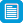# Search results

## Refine by topic

Main topic
Specific topic
Related topic
Listed under:  Science  >  Forces and energy### Kites

In this practical activity, students will learn about types of kites, some kite history and how kites fly before making and flying a kite themselves. The activity includes a teacher guide and student worksheet.### Energy skate park

This is an interactive resource about the potential and kinetic energy changes as a skater rolls around a skate park. Students learn about conservation of energy with a skater, they can build tracks, ramps and jumps for the skater and view the kinetic energy, potential energy and friction as he moves. Students can also ...### How the Rubber Meets the Road

Lesson focuses on how engineers design tyre treads to increase safety and reliability. Students are presented with the challenge of designing a new tyre tread that will be safe when driving in rainy conditions. Student teams will design and construct a sample tread out of clay, then test and evaluate the effectiveness of ...### Balloon car challenge

In this practical activity, students design and build a balloon-powered car and race it to better understand the ideas related to rocket propulsion. The resource includes a step-by-step teacher guide and student worksheets.### Pivots and Levers

Students use this resource consisting of seven slides with diagrams, written explanation and voice-over to understand that forces can cause objects to turn about a pivot and that the turning effect of a lever depends on the force and its distance from the pivot. There is a two-question quiz and a summary slide.### Stopping Distances

Students use this resource consisting of six slides with diagrams, written explanation and voice-over to understand how to calculate the total stopping distance of a car. There is a two-question quiz and a summary slide. This resource should be used before the resource 'Factors Affecting Thinking Distance'.### Factors that Affect Braking Distance

Students use this resource consisting of ten slides with diagrams, written explanation and voice-over to understand that the braking distance of a car depends on its speed and other factors which affect the frictional forces needed to stop the car. There is a two-question quiz and a summary slide.### Sites2See – Nobel Prize physics

This page features the site nobelprize.org with information on the 2007 Nobel Prize in Physics, awarded for the discovery of Giant Magnetoresistance (GMR). Applications of this phenomenon have revolutionised techniques for retrieving data from hard disks and are considered the first real applications of nanotechnology. ...### Balanced Forces

Students use this resource consisting of five slides with diagrams, written explanation and voice-over to understand that balanced forces produce no change in the movement of an object. There is a two-question quiz and a summary slide.### Fuses and Circuit Breakers

Students use this resource consisting of five slides with diagrams, written explanation and voice-over to understand how a fuse protects a circuit and learn some uses of resettable circuit breakers. There is a two-question quiz and a summary slide.### experiMENTALS: Bouncing balls

This resource contains a materials and instruction list and brief explanation for students to observe what happens when two different sized balls are dropped independently or in vertical contact. The simple explanation relates to transfer of energy.### Coal and its uses

Find out where we get the coal that powers our homes and industries. This is a PowerPoint presentation outlining how coal is formed, mined, processed and used. All slides contain images or maps. Teachers' notes are included.### experiMENTALS: Straw propeller

This resource contains a materials and instruction list and brief explanation for students about the process of creating a propeller using two bendy straws and a pair of scissors. A simple demonstration of one of Newton's Laws.### Magnetism and Electric Current

Students use this resource consisting of nine slides with diagrams, written explanation and voice-over to understand that wires carrying an electric current produce a magnetic field and that a current in a coil produces a magnetic field pattern similar to a bar magnet. There is a two-question quiz and a summary slide.### Refraction

Students use this resource consisting of ten slides with diagrams, written explanation and voice-over to understand refraction of light and know some examples. There is a two-question quiz and a summary slide.### Magnetic Fields

Students use this resource consisting of ten slides with diagrams, written explanation and voice-over to understand that a magnetic field is a region of space where magnetic materials experience forces and learn that magnetic field line patterns show the relative strength of magnetic fields. There is a two-question quiz ...### Clockwise and Anticlockwise Moments

Students use this resource consisting of five slides with diagrams, written explanation and voice-over to understand that a moment is a turning force, which can be clockwise or anticlockwise. There is a two-question quiz and a summary slide.### Stretching Materials

Students use this resource consisting of ten slides with diagrams, written explanation and voice-over to understand that the extension on a spring is proportional to the weight stretching the spring up until the elastic limit is reached (Hooke's Law). There is a two-question quiz and a summary slide.### Plotting a Magnetic Field

Students use this resource consisting of seven slides with diagrams, written explanation and voice-over to understand how to plot the magnetic field around a bar magnet using a plotting compass. There is a two-question quiz and a summary slide.### Unbalanced Forces

Students use this resource consisting of eight slides with diagrams, written explanation and voice-over to understand that unbalanced forces change the speed or direction of movement of objects and that balanced forces produce no change in the movement of an object. There is a two-question quiz and a summary slide.